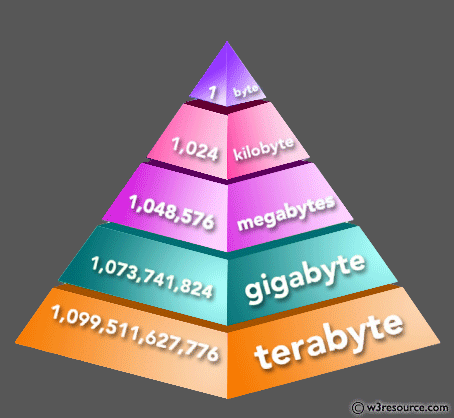﻿ Python: Get the size of an object in bytes - w3resource# Python: Get the size of an object in bytes

## Python Basic: Exercise-79 with Solution

Write a Python program to get the size of an object in bytes.

Pictorial Presentation:Sample Solution:

Python Code:

``````import sys
str1 = "one"
str2 = "four"
str3 = "three"
x = 0
y = 112
z = 122.56
print("Size of ",str1,"=",str(sys.getsizeof(str1))+ " bytes")
print("Size of ",str2,"=",str(sys.getsizeof(str2))+ " bytes")
print("Size of ",str3,"=",str(sys.getsizeof(str3))+ " bytes")
print("Size of",x,"=",str(sys.getsizeof(x))+ " bytes")
print("Size of" ,y,"="+str(sys.getsizeof(y))+ " bytes")
L = [1, 2, 3, 'Red', 'Black']
print("Size of",L,"=",sys.getsizeof(L)," bytes")
T = ("Red", [8, 4, 6], (1, 2, 3))
print("Size of",T,"=",sys.getsizeof(T)," bytes")
S = {'apple', 'orange', 'apple', 'pear'}
print("Size of",S,"=",sys.getsizeof(S)," bytes")
D = {'Name': 'David', 'Age': 6, 'Class': 'First'}
print("Size of",D,"=",sys.getsizeof(S)," bytes")
```
```

Sample Output:

```Size of  one = 52 bytes
Size of  four = 53 bytes
Size of  three = 54 bytes
Size of 0 = 24 bytes
Size of 112 =28 bytes
Size of [1, 2, 3, 'Red', 'Black'] = 104  bytes
Size of ('Red', [8, 4, 6], (1, 2, 3)) = 72  bytes
Size of {'orange', 'pear', 'apple'} = 224  bytes
Size of {'Name': 'David', 'Age': 6, 'Class': 'First'} = 224  bytes
```

Python Code Editor:

Have another way to solve this solution? Contribute your code (and comments) through Disqus.

What is the difficulty level of this exercise?

Test your Python skills with w3resource's quiz

﻿

## Python: Tips of the Day

Try-catch-else construct:

```try:
foo()
except Exception:
print("Exception occured")
else:
print("Exception didnt occur")
finally:
print("Always gets here")
```